出租广告位,需要合作请联系站长

+关注

2020-09(2)

2020-10(62)

## Python 机器学习实战 —— 无监督学习（下）

前言

4.1 KMeans 的基本原理

KMeans 均值聚类是最简单最常用的聚类算法之一，它会尝试找到代表数据区域的簇中心，并保存在 cluster_centers 属性中。再把每个数据点分配给最接近的簇中心，并把每个簇中心设置为所分配数据点的平均值。当簇中心的值根据模型运算设置不再发生变化时，算法结束。

```1 class KMeans(TransformerMixin, ClusterMixin, BaseEstimator):
2     @_deprecate_positional_args
3     def __init__(self, n_clusters=8, *, init='k-means++', n_init=10,
4                  max_iter=300, tol=1e-4, precompute_distances='deprecated',
5                  verbose=0, random_state=None, copy_x=True,
6                  n_jobs='deprecated', algorithm='auto'):```
• n_clusters：int 类型，默认为8，代表生成簇中心数目
• init：选择 {’k-means++’， ‘random ’} 之一， 或者传递一个ndarray向量，代表初始化方式，默认值为 ‘k-means++’。‘k-means++’ 用一种特殊的方法选定初始聚类中发，可加速迭代过程的收敛;‘random’ 随机从训练数据中选取初始质心。如果传递的是一个ndarray，则应该形如 (n_clusters, n_features) 并给出初始质心。
• n_init：int 类型，默认值为10，用不同的聚类中心初始化值运行算法的次数，最终解是在 inertia 意义下选出的最优结果。
• max_iter: int 类型，默认值为 300 ，代表模型优化的最大迭代数。
• tol：float类型，默认值为 1e-4 ，代表求解方法精度
• precompute_distances:  可选值 {‘auto’，True，False }, 代表预计算距离，计算速度更快但占用更多内存。‘auto’：如果 样本数乘以聚类数大于 12million 的话则不预计算距离;True：总是预先计算距离;False：永远不预先计算距离。’ deprecated ‘ 旧版本使用，新版已丢弃。
• verbose: int 类型，默认为 0，详细程度。
• random_state：默认值为None 随机数种子，推荐设置一个任意整数，同一个随机值，模型可以复现
• copy_x: bool 类型，默认值True。当 precomputing distances 生效时，将数据中心化会得到更准确的结果。如果把此参数值设为True，则原始数据不会被改变。如果是False，则会直接在原始数据上做修改并在函数返回值时将其还原。但是在计算过程中由于有对数据均值的加减运算，数据返回后，原始数据和计算前可能会有细小差别。
• n_jobs：int 类型，默认为 None, CPU 并行数。内部原理是同时进行n_init指定次数的计算时，若值为 -1，则用所有的CPU进行运算。若值为1，则不进行并行运算。若值小于-1，则用到的CPU数为(n_cpus + 1 + n_jobs)。因此如果 n_jobs值为-2，则用到的CPU数为总CPU数减1。’ deprecated ‘ 旧版本使用，新版已丢弃。
• algorithm: str 类型，{ auto，full，elkan } 三选一。full就是一般意义上的K-Means算法; elkan是使用的elkan K-Means算法; auto则会根据数据值是否是稀疏的(稀疏一般指是有大量缺失值)，来决定如何选择full 和 elkan。如果数据是稠密的，就选择elkan K-means，否则就使用普通的Kmeans算法。

• cluster_centers_:  代表簇中心 ，训练后簇中心都会被保存在此属性中
• labels_：返回数据点所属的簇标志，与 predict 方法返回值相同

4.2 KMeans 的应用场景

``` 1 def kmean_test(n):
2     #生成数据集
3     X, y = datasets.make_blobs(n_samples=100, n_features=2, random_state=1)
4     #使用KMeans模型，3个簇中心
5     kmean=KMeans(n_clusters=n)
6     model=kmean.fit_predict(X)
7     #显示运算结果
8     plt.scatter(X[:,0],X[:,1],s=50,c=model,marker='^')
9     plt.xlabel('feature0')
10     plt.ylabel('feature1')
11     #显示簇中心
12     center=kmean.cluster_centers_
13     plt.scatter(center[:,0],center[:,1],s=200,color='red',marker='.')
14     #显示簇边界
15     radii=[cdist(X[model==i],[center]).max() for i,center in enumerate(center)]
18     plt.show()
19
20 if __name__=='__main__':
21     kmean_test(3)
22     kmean_test(5)```

3 簇中心运行结果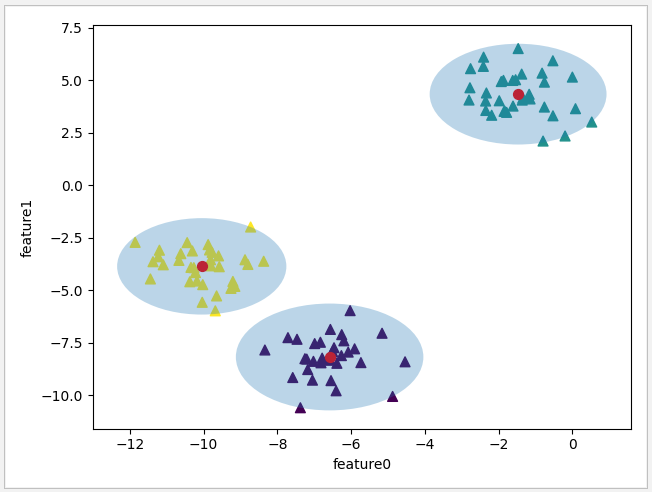5 簇中心运行结果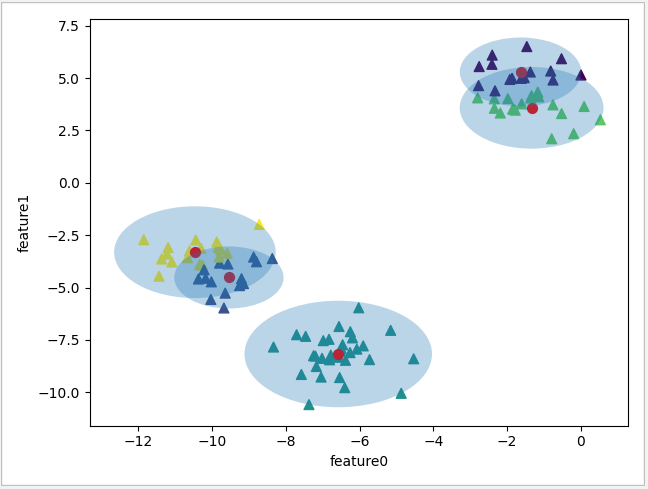``` 1 def kmean_test():
2     # 输入测试数据
4     #使用KMeans模型，10个簇中心
5     kmean=KMeans(10)
6     #训练数据
7     model=kmean.fit_predict(digits.data)
8     #计算匹配度
9     labels=np.zeros_like(model)
10     for i in range(10):
13     #计算准确率
14     acc=accuracy_score(digits.target,labels)
15     print(acc)```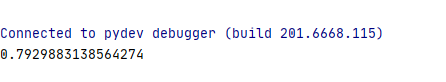``` 1 def kmean_test():
2     # 输入测试数据
4     #使用t-SNE 模型进行训练
5     tsne=TSNE()
6     model0=tsne.fit_transform(digits.data)
7     #使用KMeans模型进行训练
8     kmean=KMeans(10)
9     model1=kmean.fit_predict(model0)
10     #计算匹配度
11     labels=np.zeros_like(model1)
12     for i in range(10):
15     #计算准确率
16     acc=accuracy_score(digits.target,labels)
17     print(acc)```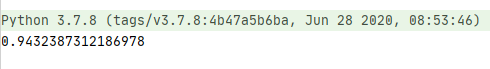4.3 通过核转换解决 KMeans 的非线性数据问题

``` 1 def kmean_test(n):
2     #生成数据集
3     X, y = datasets.make_moons(n_samples=300, noise=0.04,random_state=1)
4     #使用KMeans模型，n个簇中心
5     kmean=KMeans(n_clusters=n)
6     model=kmean.fit_predict(X)
7     #显示运算结果
8     plt.scatter(X[:,0],X[:,1],s=50,c=model,marker='^')
9     plt.xlabel('feature0')
10     plt.ylabel('feature1')
11     #显示簇中心
12     center=kmean.cluster_centers_
13     plt.scatter(center[:,0],center[:,1],s=200,color='red',marker='.')
14     plt.show()
15
16 if __name__=='__main__':
17     kmean_test(2)
18     kmean_test(10)```

n_clusters 为 2 时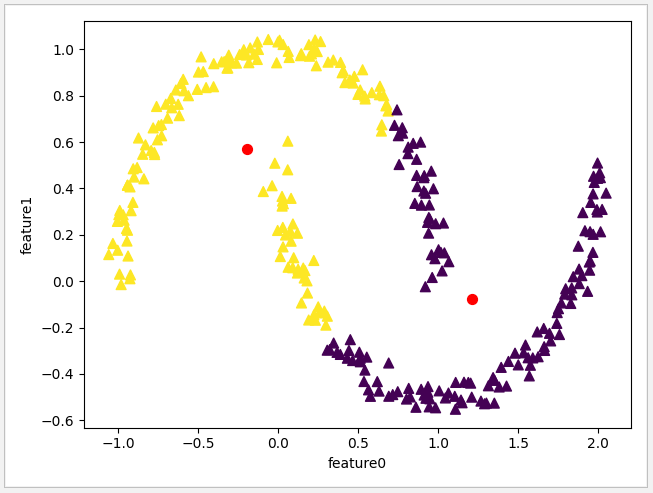n_clusters 为 10 时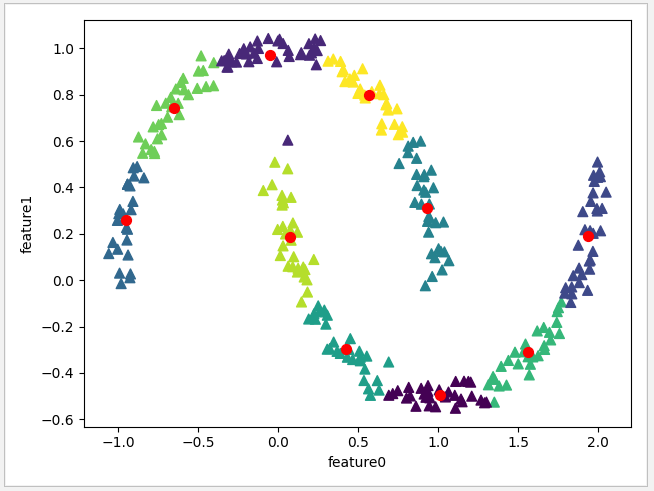```1 class SpectralClustering(ClusterMixin, BaseEstimator):
2     @_deprecate_positional_args
3     def __init__(self, n_clusters=8, *, eigen_solver=None, n_components=None,
4                  random_state=None, n_init=10, gamma=1., affinity='rbf',
5                  n_neighbors=10, eigen_tol=0.0, assign_labels='kmeans',
6                  degree=3, coef0=1, kernel_params=None, n_jobs=None,
7                  verbose=False):```
• n_clusters：int 类型，默认为 8，代表生成簇中心数目
• eigen_solver: {'arpack', 'lobpcg', 'amg','None'} 之一，默认为 None ,代表特征值求解的策略 。
• n_components：int, 默认值为 None。int 时则是直接指定特征维度数目，此时 n_components是一个大于等于 1 的整数。当使用默认值，即不输入n_components，此时n_components=min(样本数，特征数)。
• random_state：int 类型，默认值为None， 随机数种子，推荐设置一个任意整数，同一个随机值，模型可以复现。
• n_init: int 类型，默认为值 10，当 assign_labels 为 ’kmeans' 时，指定聚类中心初始化值运行算法的次数。
• gamma：float 类型，默认值为1.0，指高斯核函数的中心值。如果用k近邻法，则此参数无用。
• affinity：str 类型 {’rbf','nearest_neighbors','precomputed' , 'precomputed_nearest_neighbors'} 之一 或 callable，默认值为 ‘rbf' ，用于设定构造矩阵的方法。  ‘ rbf ' 指使用径向基函数构造矩阵(RBF)高斯内核 ; 'nearest_neighbors' 指使用 k近邻算法来构造内核; 'precomputed' 是将``X``解释为一个预先计算的亲和力矩阵，较大值表示实例之间的相似性更大; 'precomputed_nearest_neighbors': 将``X``解释为稀疏图，从每个实例的``n_neighbors``的最近邻居构造一个二进制亲和矩阵。
• n_neighbors：int 类型，默认值为10。当 affinity 设定为 ‘nearest_neighbors’ 使用 k 近邻内核时的邻居数量，当使用 ‘rbf’ 高斯内核时无效。
• eigen_tol：float 类型，默认值为 0.0。  当`eigen_solver='arpack' 时候，设定拉普拉斯矩阵特征分解的停止判断依据。
• assign_labels：str 类型 {‘kmeans’, ‘discretize’} 之一，默认值为 ‘kmeans'，用于指定分配标签的策略 。’kmeans‘ 是较常用的策略，初始化时灵敏性强。 ‘discretize’ 是随机初始化的另一种策略，灵敏性较弱。
• degree: int 类型，默认值为 3，当 eigen_solver 为 'arpack' 时，设定拉普拉斯矩阵特征分解的停止判据依据。
• coef0:  float参数 默认为1.0，核函数中的独立项，控制模型受高阶多项式的影响程度, 只有对内核为 ‘poly’和‘sigmod’ 的核函数有用
• kernel_params:   dict 类型 或者 str 类型，默认值为 None ,内核的参数。
• n_job：int 类型，默认值为 None 。当 affinity 为 ‘nearest_neighbors’ 和 ‘precomputed_nearest_neighbors’ 指定 CPU 并行数, 为 -1 指运行所有 CPU, 为 None 时只运行1个CPU。
• verbose: int 类型，默认为 0，详细程度。

``` 1 def kmean_test():
2     #生成数据集
3     X, y = datasets.make_moons(n_samples=300, noise=0.04,random_state=1)
4     #使用SpectralClustering评估器
5     spectral=SpectralClustering(n_clusters=2,affinity='nearest_neighbors',assign_labels='kmeans')
6     model=spectral.fit_predict(X)
7     #显示运算结果
8     plt.scatter(X[:,0],X[:,1],s=50,c=model,marker='^')
9     plt.xlabel('feature0')
10     plt.ylabel('feature1')
11     plt.show()```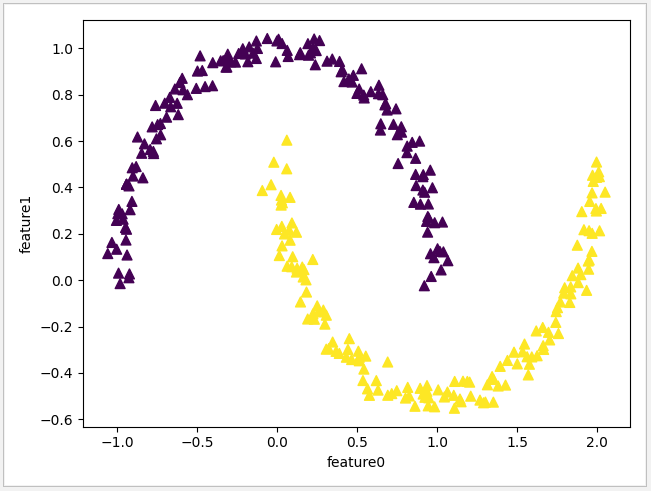5.1 GMM 的基本原理```1 class GaussianMixture(BaseMixture):
2     @_deprecate_positional_args
3     def __init__(self, n_components=1, *, covariance_type='full', tol=1e-3,
4                  reg_covar=1e-6, max_iter=100, n_init=1, init_params='kmeans',
5                  weights_init=None, means_init=None, precisions_init=None,
6                  random_state=None, warm_start=False,
7                  verbose=0, verbose_interval=10):```
• n_components: int 类型，默认为1，设定混合高斯模型个数
• covariance_type: str 类型 {‘full’,‘tied’, ‘diag’, ‘spherical ’} 之一，选择四种协方差类型，默认值为 ‘full’ 完全协方差矩阵。full: 对应完全协方差矩阵（元素都不为零), 每个分量都有各自的一般协方差矩阵 ;  tied: 相同的完全协方差矩阵（HMM会用到）所有的分量都共享相同的一般协方差矩阵; ’ diag': 对角协方差矩阵（非对角为零，对角不为零）每个分量都有各自的对角协方差矩阵 ; ‘spherical': 球面协方差矩阵（非对角为零，对角完全相同，球面特性），每个组件都有它自己的单一方差。
• tol：float 类型，默认为1e-3，EM迭代停止阈值。
• reg_covar: float 类型，默认为 1e-6，协方差对角非负正则化，保证协方差矩阵均为正。
• max_iter: int 类型，默认值 100，最大迭代次数。
• n_init: int 类型，默认为1，初始化次数，指定聚类中心初始化值运行算法的次数。
• init_params: str 类型，{‘kmeans’, ‘random’} 之一，默认值 kmeans ，初始化参数实现方式
• weights_init: array 类型 (n_components, ) ，默认为 None ，用户提供的初始权重，若为空时，则使用 init _params 方法进行初始化。
• means_init: array 类型 (n_components, n_features), 默认为 None ，用户提供的初始权重，若为空时，则使用 init _params 方法进行初始化。
• precisions_init: array 类型 ，默认为 None ，用户提供的初始权重，若为空时，则使用 init _params 方法进行初始化。当 covariance_type 为 full 则为 (n_components, n_features, n_features) 格式;  'diag' 时格式为 (n_components, n_features) ; 'tied' 时格式为 (n_features, n_features);  'spherical' 时格式为 (n_components, )
• random_state :int 类型，默认值为None， 随机数种子，推荐设置一个任意整数，同一个随机值，模型可以复现。
• warm_start : bool 类型，默认为 False，若为True，则fit（）调用会以上一次fit（）的结果作为初始化参数，适合相同问题多次fit的情况，能加速收敛。
• verbose :int 类型，默认为0，使能迭代信息显示，可以为1或者大于1（显示的信息不同）
• verbose_interval :int 类型，默认10次，与 verbose 挂钩，若使能迭代信息显示，设置多少次迭代后显示信息。

• weights_：array, 混合条件的权重
• means_：array, 均值
• covariances_:   array 协方差阵
• converged_:  bool 是否收敛

• predict_proba（X）：返回 [n_samples，n_clusters]，预测给定的数据点属于某个簇的概率
• predict_proba（） ：预测所有数据点属于某个簇的概率
• score_samples（X）：计算某个数据点样本的属于某个簇的加权对数概率

``` 1 def draw_ellipse(position, covariance, ax=None, **kwargs):
2     # 计算图形边界
3     if covariance.shape == (2, 2):
4         U, s, Vt = np.linalg.svd(covariance)
5         angle = np.degrees(np.arctan2(U[1, 0], U[0, 0]))
6         width, height = 2 * np.sqrt(s)
7     else:
8         angle = 0
9         width, height = 2 * np.sqrt(covariance)
10     # 画图
11     for nsig in range(1, 4):
12         ax.add_patch(Ellipse(position, nsig * width, nsig * height,
13                              angle, **kwargs))
14
15 def gmm_test():
16     #测试数据集
17     X, y = datasets.make_blobs(n_samples=100,centers=4,n_features=2,random_state=1)
18     #修改数据点间距
19     rng=np.random.RandomState(12)
20     X1=np.dot(X,rng.randn(2,2))
21     fig,axes=plt.subplots(1,1)
22     #使用GMM模型
23     gmm=GaussianMixture(n_components=4,random_state=28)
24     model=gmm.fit_predict(X1)
25     #显示运算结果
26     plt.scatter(X1[:,0],X1[:,1],s=50,c=model,marker='^')
27     plt.xlabel('feature0')
28     plt.ylabel('feature1')
29     #显示簇边界
30     n=0.2/gmm.weights_.max()
31     for mean,covar,weight in zip(gmm.means_,gmm.covariances_,gmm.weights_):
32         draw_ellipse(mean,covar,ax=axes,alpha=weight*n)
33     plt.show()
34
35 if __name__=='__main__':
36     gmm_test()```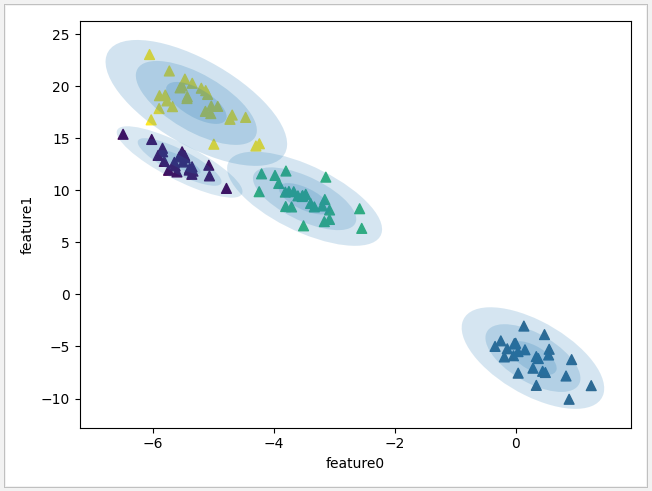Agglomerative 凝聚聚类算法首先会声明每个点都是一个簇，然后合并两个最相似的簇，直到满足设定条件准则为止。这个准则可以通过 linkage 参数确定，linkage 为 { 'ward', 'complete', 'average', 'single' } 之一，下面将会详细说明。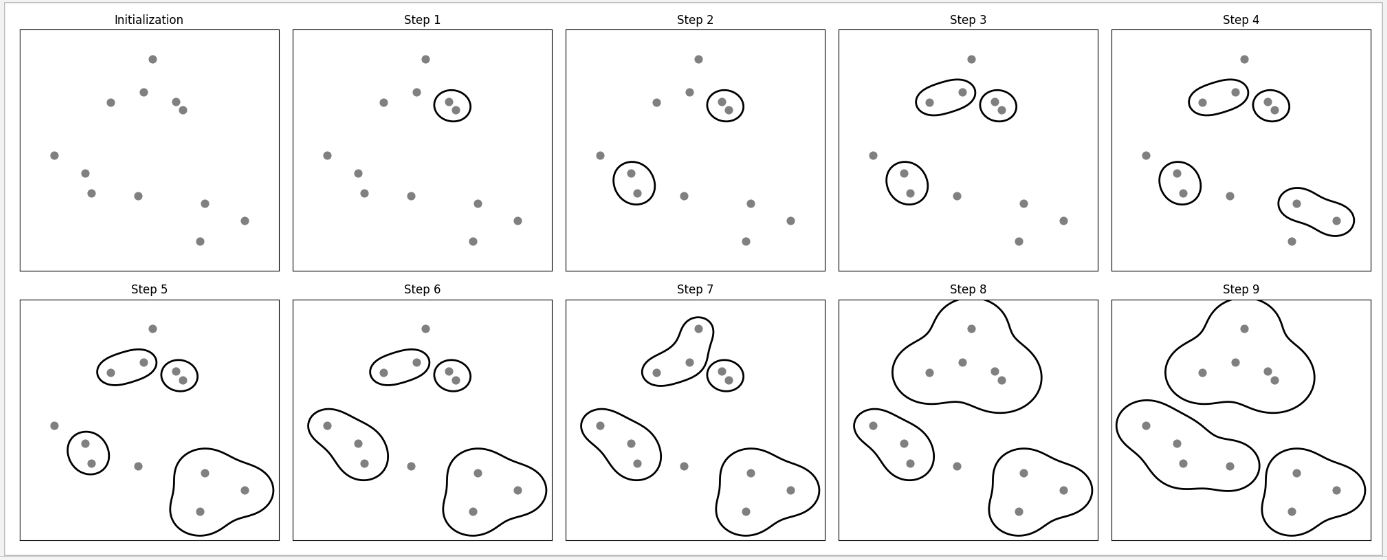构造函数

```1 class AgglomerativeClustering(ClusterMixin, BaseEstimator):
2     @_deprecate_positional_args
3     def __init__(self, n_clusters=2, *, affinity="euclidean",
4                  memory=None,
5                  connectivity=None, compute_full_tree='auto',
7                  compute_distances=False):```
• n_clusters：int 类型，默认值为2，指定最终分类簇的数量
• affinity：str 类型，{ ’euclidean’，’l1’，’l2’，’mantattan’，’cosine’，’precomputed’ }之一，默认值为 ’euclidean’  ，作用是选择一个计算距离的可调用对象。当 linkage=’ward’，affinity 只能为’euclidean’
• memory：str 类型，默认值为 None, 用于缓存计算结果的输出，默认不作任何缓存。如果设定字符串路径，则它会缓存到对应的路径。
• connectivity：array-like 或 callable 类型，默认为 None，用于指定连接矩阵，临近的数据将使用相同的结构。
• compute_full_tree：bool 类型，默认值为 auto，当训练了 n_clusters后，训练过程就会停止，但是如果 compute_full_tree=True，则会继续训练从而生成一颗完整的树。当 distance_threshold 不为 None 时，则 compute_full_tree 必须为是 True。
• linkage：str 类型， { 'ward', 'complete', 'average', 'single' } 之一，默认值为 'ward' 一个字符串，用于指定链接算法。‘ward’：单链接 single-linkage，使所有簇中的方差 dmindmin 增加最小的簇合并; ‘complete’：全链接 complete-linkage 算法，使簇中点之间的最大距离 dmaxdmax 最小的两个簇合并 ;  'average’：均连接 average-linkage 算法，使簇中所有点之间平均距离 davgdavg  最小的两个簇合并。’single' ：新增于 0.20 版，使簇中所有点距离最小的两个簇合并。
• distance_threshold：float 类型，默认值为 None，链接距离阈值超过此设置，集群将不会合并。当使用此设置时，‘n_clusters’ 必须是 None 和 'compute_full_tree' 必须是 True 。
• compute_distances：bool 类型，默认值为 False，当不使用 distance_threshold 时，计算簇之间的距离。此功能可以用于使树状图可视化，但此计算将消耗计算机的内存。

``` 1 def agglomerative_test():
2     # 测试数据集
3     X,y=make_blobs(random_state=1)
4     # 使用 AgglomerativeClustering模型
5     agglomerative=AgglomerativeClustering(n_clusters=3)
6     # 开始运算
7     model=agglomerative.fit_predict(X)
8     # 显示簇合并结果
9     fig, axes = plt.subplots(1, 2, figsize=(10,5))
10     ax0=axes
11     ax0.scatter(X[:,0],X[:,1],s=50,c=model,marker='^')
12     # 显示树状图
13     ax1=axes
14     shc.dendrogram(shc.ward(X[::5]))
15     plt.show()```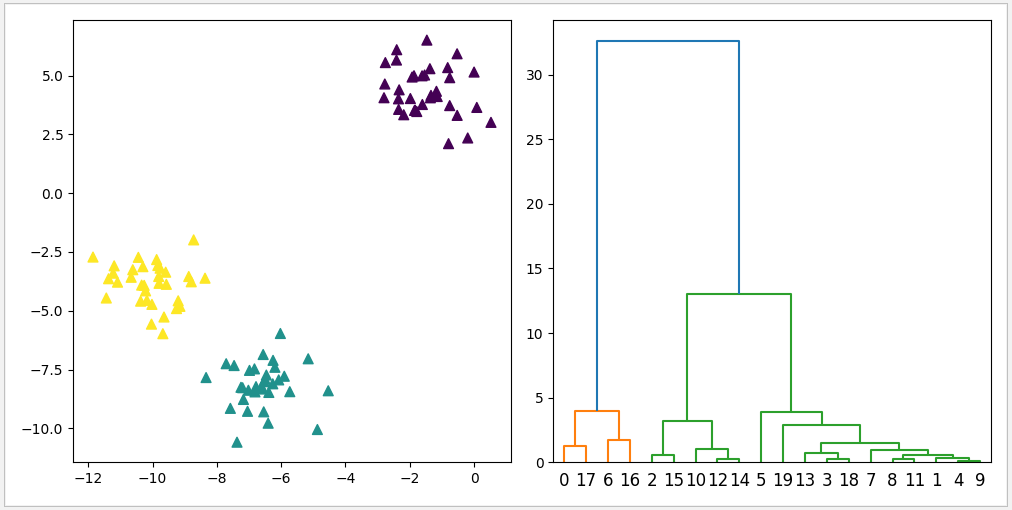DBSCAN 密度聚类是最常用的模型之一，一般用于分析形状比较复杂的簇，还可找到不属于任何簇的数据点。上面介绍到的 KMeans 模型、SpectralClustering 评估器、Agglomerative 模型都需要在建立模型前通过 n_clusters 参数预先设置簇的个数，然而在复杂的数据中，单凭简单的数据分析去正确评估簇数据是很困难的。 DBSCAN 的优点在于它不需要预先设置簇的个数，而是通过特征空间的数据点密度来区分簇。DBSCAN 最常用到的参数是 eps 和 min_samples，eps 是用于设定距离，DBSCAN 将彼此距离小于 eps 设置值的核心样本放在同一个簇。min_samples 则用于设置同一簇内数据点的最少数量，如果数据点数量少于此设置则把这此点默认为噪点 noise，数据点数量大于等于此数值时则默认一个簇。

```1 class DBSCAN(ClusterMixin, BaseEstimator):
2     @_deprecate_positional_args
3     def __init__(self, eps=0.5, *, min_samples=5, metric='euclidean',
4                  metric_params=None, algorithm='auto', leaf_size=30, p=None,
5                  n_jobs=None):```
• esp：float 类型，默认值为 0.5，用于设定距离，DBSCAN 将彼此距离小于 eps 设置值的核心样本放在同一个簇。
• min_samples: int 类型，默认值为5，用于设置同一簇内数据点的最少数量，如果数据点数量少于此设置则把这此点默认为噪点 noise，数据点数量大于等于此数值时则默认一个簇。
• metric：string 或 callable 类型，['cityblock', 'cosine', 'euclidean', 'l1', 'l2', 'manhattan'] 之一，默认值为 ‘euclidean’, 用于定义计算数据点之间的距离时使用的度量。使用欧式距离 “euclidean”; 使用曼哈顿距离 “manhattan”;
• metric_params: dict 类型，默认值为 None，根据 metric 选择填入相关参数。
• algorithm：str 类型，{'auto', 'ball_tree', 'kd_tree', 'brute'} 之一，默认值为 auto，定义计算近邻数据点的方法。 ‘auto’：会在上面三种算法中做权衡，选择一个拟合最好的最优算法。‘ball_tree’  球面树搜索，数据点比较分散时可试用 ball_tree; kd_tree: kd树搜索，数据点分布比较均匀时效率较高; ‘brute’：暴力搜索;
• leaf_size：int 类型，默认为30，定义停止建立子树的叶子节点数量的阈值。 最近邻搜索算法参数，为使用KD树或者球树时， 这个值越小，则生成的KD树或者球树就越大，层数越深，建树时间越长，反之，则生成的KD树或者球树会小，层数较浅，建树时间较短。
• p: 最近邻距离度量参数。只用于闵可夫斯基距离和带权重闵可夫斯基距离中p值的选择，p=1为曼哈顿距离， p=2为欧式距离。如果使用默认的欧式距离不需要管这个参数。
• n_jobs：CPU 并行数，默认为None，代表1。若设置为 -1 的时候，则用所有 CPU 的内核运行程序。

``` 1 def dbscan_test(eps,title):
2     # 测试数据集
3     X,y=datasets.make_blobs(random_state=1)
4     # 使用DBSCAN，输入eps参数
5     dbscan=DBSCAN(eps=eps)
6     model=dbscan.fit_predict(X)
7     # 显示测试后结果
8     plt.scatter(X[:,0],X[:,1],c=model,s=50,marker='^')
9     plt.xlabel('feature0')
10     plt.ylabel('feature1')
11     plt.title(title)
12     plt.show()
13
14 if __name__=='__main__':
15     dbscan_test(0.5,'EPS:0.5 ')
16     dbscan_test(1,'EPS 1')
17     dbscan_test(1.5,'EPS 1.5')```

eps = 0.5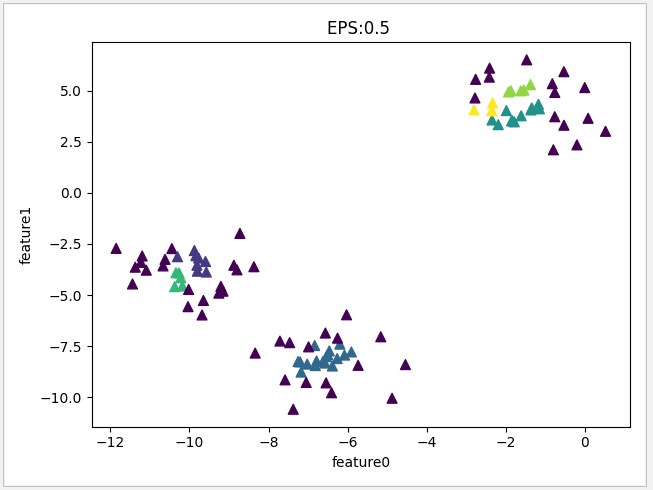eps = 1.0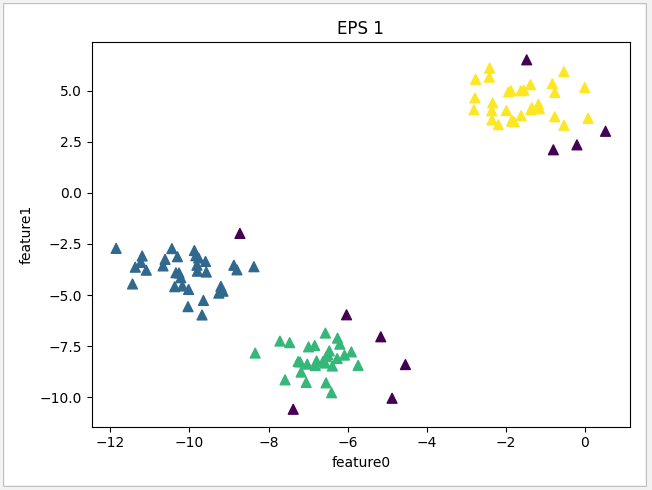eps = 1.5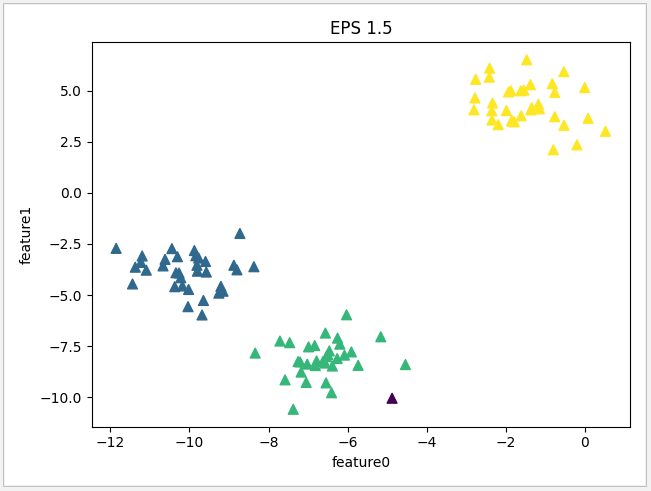``` 1 def dbscan_test(min,title):
2     # 测试数据集
3     X,y=datasets.make_blobs(random_state=1)
4     # 使用DBSCAN，输入eps,min_samples参数
5     dbscan=DBSCAN(eps=1.0,min_samples=min)
6     model=dbscan.fit_predict(X)
7     # 显示测试后结果
8     plt.scatter(X[:,0],X[:,1],c=model,s=50,marker='^')
9     plt.xlabel('feature0')
10     plt.ylabel('feature1')
11     plt.title(title)
12     plt.show()
13
14 if __name__=='__main__':
15     dbscan_test(20,'MIN SAMPLES 20')
16     dbscan_test(10,'MIN SAMPLES 10')
17     dbscan_test(5,'MIN SAMPLES 5')```

min_samples = 20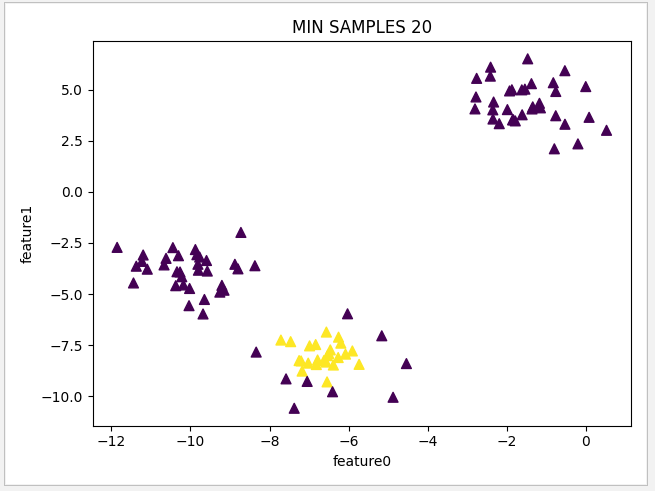min_samples = 10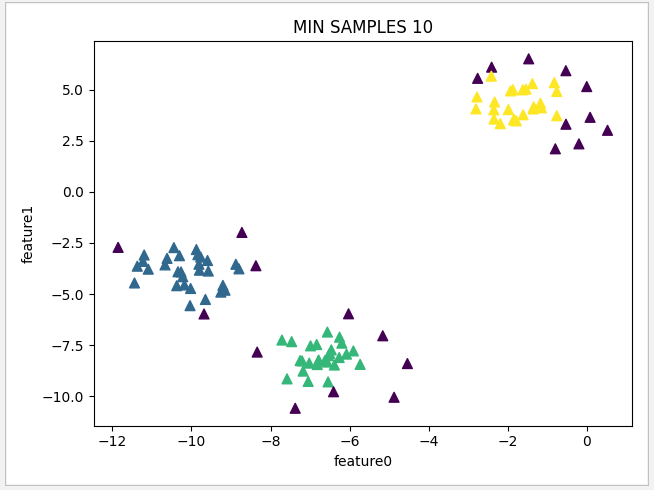min_samples = 5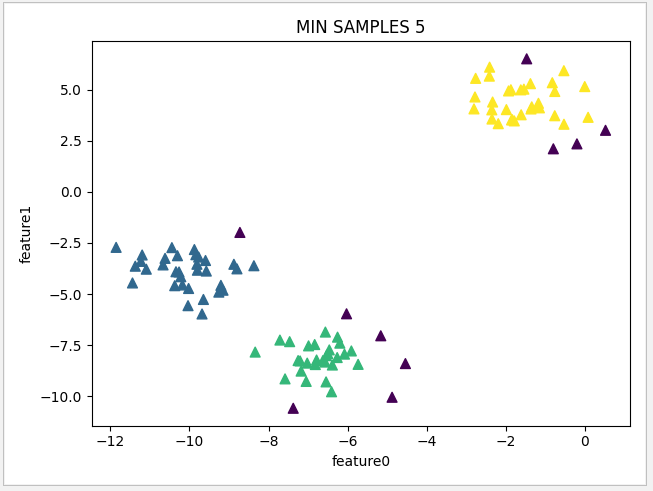``` 1 def dbscan_test(title):
2     # 测试数据集
3     X, y = datasets.make_moons(n_samples=300, noise=0.04,random_state=1)
4     # 使用DBSCAN，输入eps,min_samples参数
5     dbscan=DBSCAN(eps=0.4,min_samples=30)
6     model=dbscan.fit_predict(X)
7     # 显示测试后结果
8     plt.scatter(X[:,0],X[:,1],c=model,s=50,marker='^')
9     plt.xlabel('feature0')
10     plt.ylabel('feature1')
11     plt.title(title)
12     plt.show()
13
14 if __name__=='__main__':
15     dbscan_test('DBSCAN')```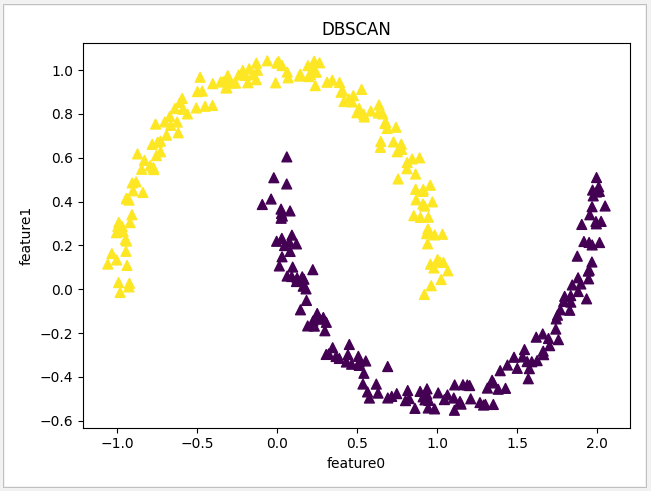``` 1 def dbscan_test():
2     # 测试数据集
3     person = datasets.fetch_lfw_people()
4     # 使用PCA模型把特征降至60
5     pca=PCA(0.95,whiten=True,random_state=1)
6     pca_model=pca.fit_transform(person.data)
7     # 使用DBSCAN，输入eps,min_samples参数
8     dbscan=DBSCAN(eps=23,min_samples=3)
9     model=dbscan.fit_predict(pca_model)
10     # 显示测试后结果
11     fig,axes=plt.subplots(5, 5, figsize=(25,25))
12     # 获取噪点的特征成分图
13     noise=pca_model[model==-1]
14     # 输出噪点数量
15     print(str.format('noise len is {0}'.format(len(noise))))
16     for componemt,ax in zip(noise,axes.ravel()):
17         # 获取噪点提取成分后还原图
18         image=pca.inverse_transform(componemt)
19         ax.imshow(image.reshape(62, 47), cmap = 'viridis')
20     plt.show()    ```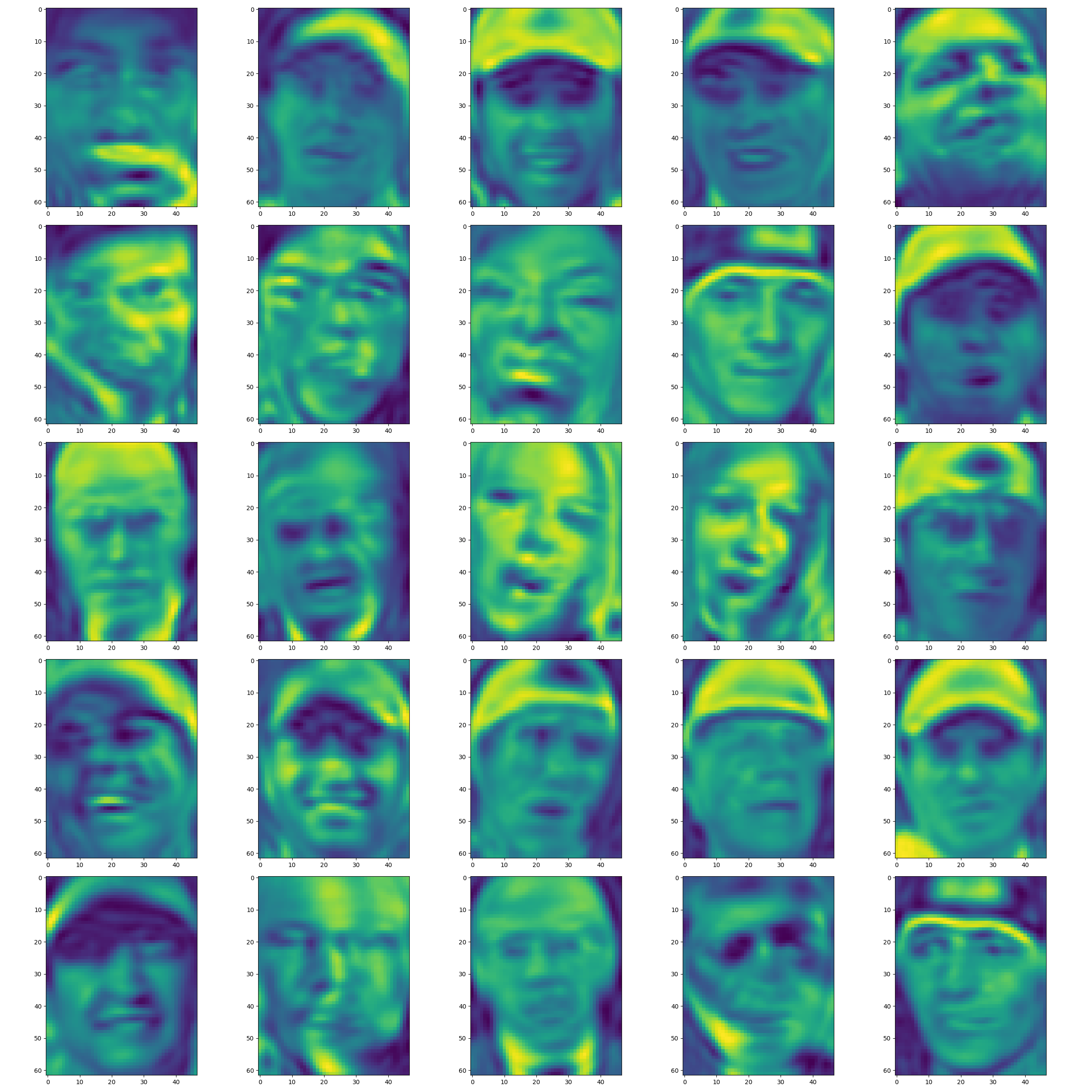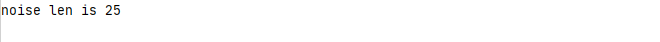Python 机器学习实战 —— 监督学习（上）

Python 机器学习实战 —— 监督学习（下）

Python 机器学习实战 —— 无监督学习（上）

Python 机器学习实战 —— 无监督学习（下）

5 0

pdf(new) 更多>